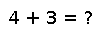# zip

``````..  zip(iters...)

For a set of iterable objects, returns an iterable of tuples, where the ``i``\ th tuple contains the ``i``\ th component of each input iterable.

Note that :func:`zip` is its own inverse: ``collect(zip(zip(a...)...)) == collect(a)``.``````

## Examples

``````julia> a = [1,2,3];
julia> b = [4,5,6,7];
julia> foo = zip(a, b);
julia> for i in foo
print(i)
end
(1,4)(2,5)(3,6)
julia> # 7 not paired``````

Checking you are not a robot: